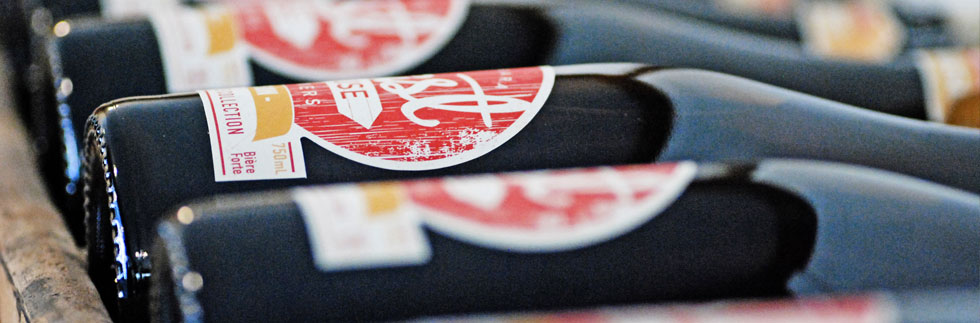Today we are open from
11:00am until 9:00pm
Niagara-on-the-Lake
L0S 1J0
289.868.9627
info@oasthousebrewers.ca

## Subscribe to our mailing list

* indicates required

### Beers7.0%
ALC
20
IBU
750
ml
• h
7.0%
ALC
20
IBU
750
ml
• h
7.9%
ALC
28
IBU
750
ml
• h
5.5%
ALC
18
IBU
750
ml
• h
7.5%
ALC
35
IBU
750
ml
• h
6.4%
ALC
7
IBU
750
ml
• h
6.2%
ALC
7
IBU
750
ml
• h

6.0%
ALC
55
IBU
1900
ml
• e
• f
• g
6.5%
ALC
70
IBU
1900
ml
• e
• f
• g
5.0%
ALC
16
IBU
1900
ml
• e
• f
• g
6.5%
ALC
16
IBU
1900
ml
• e
• f
• g
5%
ALC
30
IBU
1900
ml
• e
• f
• g
6.9%
ALC
18
IBU
1900
ml
• e
• f
• g
5.3%
ALC
20
IBU
1900
ml
• e
• f
• g
6.0%
ALC
18
IBU
1900
ml
• e
• f
• g
6.0%
ALC
25
IBU
1900
ml
• e
• f
• g
4.2%
ALC
25
IBU
1900
ml
• e
• f
• g
6.5%
ALC
45
IBU
1900
ml
• e
• f
• g
5.5%
ALC
18
IBU
1900
ml
• e
• f
• g
5.6%
ALC
25
IBU
1900
ml
• e
• f
• g
6.1%
ALC
70
IBU
1900
ml
• e
• f
• g
6.0%
ALC
50
IBU
1900
ml
• e
• f
• g

4.8%
ALC
17
IBU
473
ml
• a
• c
• e
• f
• g
4.9%
ALC
8
IBU
473
ml
• a
• c
• e
• f
• g
5.5%
ALC
30
IBU
473
ml
• a
• c
• e
• f
• g
5.0%
ALC
18
IBU
473
ml
• a
• c
• e
• f
• g
4.7%
ALC
7
IBU
473
ml
• a
• c
• e
• f
• g
6.1%
ALC

IBU
473
ml
• a
• c
• e
• f
• g
5.4%
ALC
18
IBU
473
ml
• a
• c
• e
• f
• g
5.3%
ALC
20
IBU
473
ml
• a
• c
• e
• f
• g
7.1%
ALC
30
IBU
473
ml
• a
• c
• e
• f
• g
10.4%
ALC
55
IBU
473
ml
• a
• c
• e
• f
• g
10.1%
ALC
25
IBU
473
ml
• a
• c
• e
• f
• g
5.0%
ALC
16
IBU
473
ml
• a
• c
• e
• f
• g

5.0%
ALC
16
IBU
473
ml
• a
• c
• e
• f
• g
5.3%
ALC
20
IBU
473
ml
• a
• c
• e
• f
• g